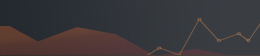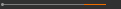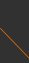Sponsored by[ Events ]

﻿
Activity Search
Sort out
 select all Seminars and Talks Conference and Workshop Lectures and Courses ElseField
 select all Number Theory and Representation Theory Algebraic Geometry Differential Geometry and Geometrical Analysis Differential Equations and Stochastic Analysis Scientific Computing Interdisciplinary Studies Laboratory of Data Science Harmonic Analysis OthersYear
 select 2015 2016 2017 2018 2019 2020 2021NCTS PDE & Analysis Seminar14:20 - 15:10, March 11, 2020 (Wednesday)
R304, Astronomy-Mathematics Building, NTU
(台灣大學天文數學館 304室)
Uniqueness and Global Convergence for a Discrete Inverse Coefficient Problem
Bastian von Harrach (Goethe-University Frankfurt)

## This seminar is cancelled.

Abstract:

We derive a simple criterion that ensures uniqueness, Lipschitz stability and global convergence of Newton's method for the finite dimensional zero-finding problem of a continuously differentiable, pointwise convex and monotonic function. Our criterion merely requires to evaluate the directional derivative of the forward function at finitely many evaluation points and for finitely many directions.

We then demonstrate that this result can be used to prove uniqueness, stability and global convergence for an inverse coefficient problem with finitely many measurements. We consider the problem of determining an unknown inverse Robin transmission coefficient in an elliptic PDE. Using a relation to monotonicity and localized potentials techniques, we show that a piecewise-constant coefficient on an a-priori known partition with a-priori known bounds is uniquely determined by finitely many boundary measurements and that it can be uniquely and stably reconstructed by a globally convergent Newton iteration. We derive a constructive method to identify these boundary measurements, calculate the stability constant and give a numerical example.(C) 2019 National Center for Theoretical Sciences